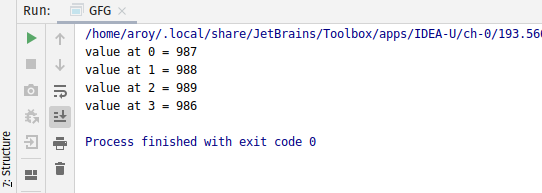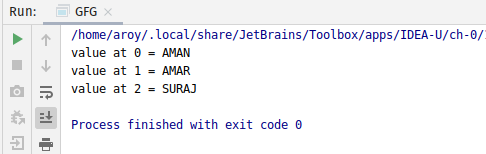# AtomicReferenceArray getPlain() method in Java with Examples

The getPlain() method of a AtomicReferenceArray class is used to return the value of the element at index i for this AtomicReferenceArray object with with memory semantics of reading as if the variable was declared non-volatile.

Syntax:

```public final E getPlain(int i)
```

Parameters: This method accepts the index i to get the value.

Return value: This method returns current value at index i.

Below programs illustrate the getPlain() method:
Program 1:

 `// Java program to demonstrate ` `// AtomicReferenceArray.getPlain() method ` ` `  `import` `java.util.concurrent.atomic.*; ` ` `  `public` `class` `GFG { ` `    ``public` `static` `void` `main(String[] args) ` `    ``{ ` ` `  `        ``// create an atomic reference array ` `        ``// object which stores Integer. ` `        ``AtomicReferenceArray array ` `            ``= ``new` `AtomicReferenceArray(``5``); ` ` `  `        ``// set some value in array ` `        ``array.set(``0``, ``987``); ` `        ``array.set(``1``, ``988``); ` `        ``array.set(``2``, ``989``); ` `        ``array.set(``3``, ``986``); ` ` `  `        ``// get and print the value ` `        ``// using getPlain method ` `        ``for` `(``int` `i = ``0``; i < ``4``; i++) { ` ` `  `            ``int` `value = array.getPlain(i); ` `            ``System.out.println(``"value at "` `                               ``+ i + ``" = "` `                               ``+ value); ` `        ``} ` `    ``} ` `} `

Output:Program 2:

 `// Java program to demonstrate ` `// AtomicReferenceArray.getPlain() method ` ` `  `import` `java.util.concurrent.atomic.*; ` ` `  `public` `class` `GFG { ` `    ``public` `static` `void` `main(String[] args) ` `    ``{ ` ` `  `        ``// create a array of Strings ` `        ``String[] names ` `            ``= { ``"AMAN"``, ``"AMAR"``, ``"SURAJ"` `}; ` ` `  `        ``// create an atomic reference object. ` `        ``AtomicReferenceArray array ` `            ``= ``new` `AtomicReferenceArray(names); ` ` `  `        ``// get and print the value ` `        ``// using getPlain method ` `        ``for` `(``int` `i = ``0``; i < array.length(); i++) { ` ` `  `            ``String value = array.getPlain(i); ` `            ``System.out.println(``"value at "` `                               ``+ i + ``" = "` `                               ``+ value); ` `        ``} ` `    ``} ` `} `

Output:Attention reader! Don’t stop learning now. Get hold of all the important Java and Collections concepts with the Fundamentals of Java and Java Collections Course at a student-friendly price and become industry ready.

My Personal Notes arrow_drop_upCheck out this Author's contributed articles.

If you like GeeksforGeeks and would like to contribute, you can also write an article using contribute.geeksforgeeks.org or mail your article to contribute@geeksforgeeks.org. See your article appearing on the GeeksforGeeks main page and help other Geeks.

Please Improve this article if you find anything incorrect by clicking on the "Improve Article" button below.

Article Tags :
Practice Tags :

Be the First to upvote.

Please write to us at contribute@geeksforgeeks.org to report any issue with the above content.